﻿ 考虑多控制断面流量需求的湘江水库群优化调度图研究 Derivations of the Xiang River Reservoirs Operation Rule Curves Considering Flow Demand in Multiple Control Sections

Journal of Water Resources Research
Vol. 08  No. 04 ( 2019 ), Article ID: 31463 , 10 pages
10.12677/JWRR.2019.84042

Derivations of the Xiang River Reservoirs Operation Rule Curves Considering Flow Demand in Multiple Control Sections

Zhikai Yang1, Yini Liu1, Xiaoqing Zhou2, Pan Liu1*, Bo Ming1, Wei Huang2

1State Key Laboratory of Water Resources and Hydropower Engineering Science, Wuhan University, Wuhan HubeiReceived: Jun. 24th, 2019; accepted: Jul. 16th, 2019; published: Jul. 26th, 2019ABSTRACT

The construction of cascade reservoirs has greatly promoted the development and utilization of water resources in the Xiang River. However, a single reservoir operating mode is adopted between the cascades, and the various utilities of the reservoirs cannot be fully exploited. Based on the joint operation of nine hydropower stations in the Xiang River, the main objective is ensuring the ecological flow demand of control sections, and multiple targets such as water supply, irrigation and power generation are also taken into account. A multi-objective optimization model for the Xiang River’s cascade reservoirs is established. The cuckoo search algorithm is used to optimize the multi-objective operating curve rules, so that the ecological guarantee rate of each control section and the downstream Changsha integrated hub is above 99%. Meanwhile, the water supply guarantee rate, the irrigation guarantee rate and the power generation guarantee rate of the cascade reservoirs are as far as possible to meet the actual production.

Keywords:Operating Rule Curves, Comprehensive Utilization Reservoir, Multi-Reservoir Operation, Cuckoo Search Algorithm, The Xiang River

1武汉大学水资源与水电工程科学国家重点实验室，湖北 武汉

2湖南省水利水电勘测设计研究总院，湖南 长沙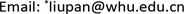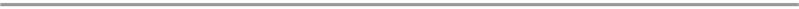1. 引言

2. 研究方法

2.1. 供水规则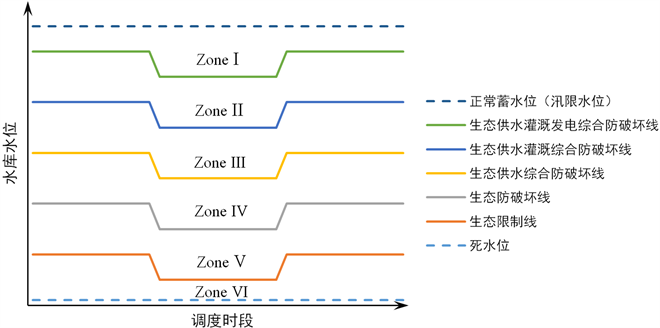Figure 1. Example of reservoir operating rule curves for comprehensive utilizationTable 1. Description of scheduling decision-making in decision-making area of comprehensive utilization of reservoir operating rule curves

2.2. 模型建立

2.2.1. 目标函数

1) 水库群生态供水保证率最大化，即

$MaxR1\left(T\right)=Max\underset{t=1}{\overset{T}{\sum }}\underset{m=1}{\overset{M}{\sum }}\frac{#\left[{Q}_{m}^{E}\left(t\right)\ge {Q}_{m}^{ED}\right]}{T}$ (1)

2) 水库群生活供水保证率最大化，即

$MaxR2\left(T\right)=Max\underset{t=1}{\overset{T}{\sum }}\underset{k1=1}{\overset{K1}{\sum }}\frac{#\left[{Q}_{k1}^{L}\left(t\right)\ge {Q}_{k1}^{LD}\right]}{T}$ (2)

3) 水库群灌溉供水保证率最大化，即

$MaxR3\left(T\right)=Max\underset{t=1}{\overset{T}{\sum }}\underset{k2=1}{\overset{K2}{\sum }}\frac{#\left[{Q}_{k2}^{I}\left(t\right)\ge {Q}_{k2}^{ID}\left(t\right)\right]}{T}$ (3)

4) 水库群发电保证率最大化，即

$MaxR4\left(T\right)=Max\underset{t=1}{\overset{T}{\sum }}\underset{k3=1}{\overset{K3}{\sum }}\frac{#\left[{P}_{k3}\left(t\right)\ge {P}_{k3}^{firm}\right]}{T}$ (4)

5) 水库群总发电量最大化，即

$MaxP\left(T\right)=Max\underset{t=1}{\overset{T}{\sum }}\underset{k3=1}{\overset{K3}{\sum }}{P}_{k3}\left(t\right)\cdot \Delta t$ (5)

2.2.2. 约束条件

1) 水量平衡约束

${V}_{k}\left(t\right)={V}_{k}\left(t-1\right)+\left({Q}_{in}^{k}\left(t\right)-{Q}_{out}^{k}\left(t\right)\right)\Delta t$ (6)

2) 流量平衡约束

${Q}_{in}^{k+1}\left(t\right)={Q}_{out}^{k}\left(t\right)+{q}_{k}^{k+1}\left(t\right)$ (7)

3) 水库水位约束

${Z}_{\mathrm{min}}^{k}\left(t\right)\le {Z}_{n}\left(t\right)\le {Z}_{\mathrm{max}}^{k}\left(t\right)$ (8)

4) 泄流能力约束

${Q}_{out}^{k}\left(t\right)\le {Q}_{\mathrm{max}}^{k}\left(t\right)$ (9)

5) 电站出力约束

${P}^{k}\left(t\right)\le {P}_{\mathrm{max}}^{k}\left(t\right)$ (10)

6) 调度线形状约束，指各调度线不交叉

${L}_{x-1}^{k}\le {L}_{x}^{k}$ (11)

2.3. 模型求解

2.3.1. 布谷鸟搜索算法

1) 引入动态发现概率解决进化后期种群质量高，容易陷入局部最优解的问题。区别于固定发现概率，动态发现概率在搜索后期增大，以增加种群进化强度；

2) 引入自适应动态步长，在搜索初期，解集改变比例大，因此莱维飞行步长需较大以保持全局搜索，提高寻优效率。在搜索后期，新解改变越来越小，趋向于短距离搜索，精细化寻优，则莱维飞行步长自适应变小。

CS的具体计算步骤如下：

1) 随机生成N个序列，其中每个序列包括r座水库的n个关键节点时间空间信息编码，初始化种群；

2) 计算当前所有种群的适应度，并筛选出最优种群；

3) 莱维飞行更新。根据莱维飞行全局步长公式(12)更新当前鸟窝位置，计算更新后的适应度值。若更新后的子代鸟窝支配父代鸟窝，则父代鸟窝被子代鸟窝替换，反之不替换。

$\begin{array}{l}{X}_{{}^{i}}^{l+1}={X}_{{}^{i}}^{l}+\alpha \oplus L\left(\lambda \right)\\ L\left(\lambda \right)=\frac{u}{{|v|}^{1/\beta }}\left({X}_{i}^{l}-{X}_{best}^{l}\right)\end{array}$ (12)

$\begin{array}{l}u~N\left(0,{\sigma }_{u}^{2}\right),v~N\left(0,{\sigma }_{v}^{2}\right)\\ {\sigma }_{u}^{2}={\left\{\frac{\Gamma \left(1+\beta \right)\mathrm{sin}\left(\pi \beta /2\right)}{\Gamma \left[\left(1+\beta \right)/2\right]\beta {2}^{\left(\beta -1\right)/2}}\right\}}^{1/\beta },{\sigma }_{v}^{2}=1\end{array}$ (13)

${\alpha }_{0}^{l+1}={\alpha }_{0}^{l}\cdot \mathrm{exp}\left(K\left(r-T\right)\right)$ (14)

${X}_{{}^{i}}^{l+1}={X}_{{}^{i}}^{l}+{\alpha }_{0}^{l}\frac{{\sigma }_{u}^{2}\cdot u}{{|v|}^{1/\beta }}\left({X}_{i}^{l}-{X}_{best}^{l}\right)$ (15)

4) 偏好随机游动更新。根据一定发现概率 ${P}_{a}$ 随机选取一部分鸟窝，局部更新个体。生成一个随机数并与发现概率 ${P}_{a}$ 对比，若随机数小于 ${P}_{a}$ 则更新该个体。

${x}_{i}^{l+1}={x}_{i}^{l}+\gamma \cdot H\left({P}_{a}-\epsilon \right)\otimes \left[{x}_{j}^{l}-{x}_{k}^{l}\right]$ (16)

${P}_{a}=0.1\cdot \mathrm{cos}\left[\frac{\left({N}_{iter}-1\right)\pi }{2\left({N}_{final}-1\right)}\right]+0.1$ (17)

5) 计算种群适应度，记录当前最优解，进行下一轮迭代；

6) 满足迭代终止条件后，终止迭代，输出寻优结果。

2.3.2. 调度线编码

$suq\left(j,i\right)=\left[\underset{\text{time}}{\underset{︸}{\left({t}_{j,i}^{1},\cdots ,{t}_{j,i}^{n}\right)}},\underset{\text{waterlevel}}{\underset{︸}{\left({Z}_{j,i}^{1},\cdots ,{Z}_{j,i}^{n},{Z}_{j,i}^{END}\right)}}\right]$ (18)

(19)

2.3.3. 调度图模拟

3. 研究实例

3.1. 流域概况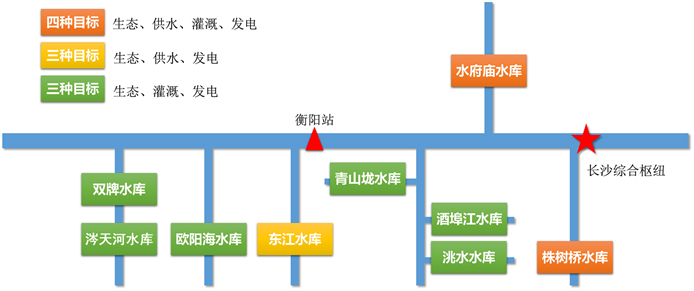Figure 2. The topological map of reservoirs in the study basin

3.2. 结果与分析

3.2.1. 调度图

3.2.2. 生态调度结果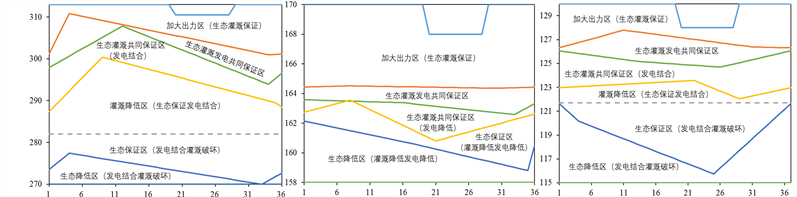Figure 3. Results of reservoir operating rule curves for comprehensive utilization in the Xiang RiverFigure 4. Results of minimum flow demand of ecological control sections and optimal operation in the Xiang River

3.2.3. 其他兴利目标调度结果

1) 对于具有供水任务的水库，东江水库的供水保证率达100%，说明所有时段的供水需求全部得到满足，供水效益较好。而对于水府庙水库，供水保证率为98.92%，满足实际要求供水保证率97%的目标。Table 2. Statistics on results of other objectives of reservoirs in the Xiang River

2) 对于具有灌溉任务的水库，涔天河水库和洮水水库的灌溉设计保证率为85%，其余六座水库的灌溉设计保证率均为90%。从表2的统计结果可知，8个有灌溉任务的水库中，有一半水库的灌溉保证率达到灌溉设计保证率的要求。由于灌溉的优先级稍低，所以较难保证所有水库的灌溉保证率都能得到满足。其中，位于上游的涔天河、双牌、欧阳海和洮水四座水库的灌溉保证率接近100%，灌溉满足情况较好。而下游的水库灌溉满足情况普遍劣于上游水库。

3) 对于流域内的所有水库，都具有发电的功能，但该功能的兴利优先级在每座水库中都是最低的。从表2中可以看出，各水库多年平均发电量多数都较小。对于发电保证率，涔天河、双牌、欧阳海、洮水和水府庙五座水库均在90%以上。由于各水库在发挥生态、供水和灌溉功能后，其发电的用水量将得到削减，发电效益在一定程度上会被削弱。但对于9座水库中装机最大的东江水库，其装机容量为560 MW，接近所有水库装机总容量的1/2。其设计多年平均发电量为9亿kWh，模拟调度结果为10.13亿kWh，发电效益良好。

4. 结语

1) 本文将布谷鸟搜索算法应用于梯级水库多目标调度图优化中，通过引入动态发现概率和自适应步长改进算法，实例结果验证了布谷鸟搜索算法在梯级水库多目标调度图优化问题中收敛速度快，具有有效性。

2) 本文通过优化调度图，保障湘江梯级9座水电站以及下游长沙综合枢纽的生态供水保证率基本均在99%以上，满足多控制断面最小生态流量目标，基本符合湘江生态供水需求。

3) 本文通过梯级水库联合调度，实现了在保障生态流量目标的基础上，尽可能发挥供水效益、灌溉效益和发电效益，充分开发梯级水库的综合职能效益，提出的调度模型具有可行性。

Derivations of the Xiang River Reservoirs Operation Rule Curves Considering Flow Demand in Multiple Control Sections[J]. 水资源研究, 2019, 08(04): 361-370. https://doi.org/10.12677/JWRR.2019.84042

1. 1. ZHOU, Y., GUO, S., CHANG, F., et al. Boosting hydropower output of mega cascade reservoirs using an evolutionary algorithm with successive approximation. Applied Energy, 2018, 228: 1726-1739. https://doi.org/10.1016/j.apenergy.2018.07.078

2. 2. 王本德, 周惠成, 卢迪. 我国水库(群)调度理论方法研究应用现状与展望[J]. 水利学报, 2016, 47(3): 337-345. WANG Bende, ZHOU Huicheng and LU Di. The state-of-the-art review in the theory research and its application of mul-ti-reservoir system operation in China. Journal of Hydraulic Engineering, 2016, 47(3): 337-345. (in Chinese)

3. 3. LIU, P., GUO, S., XU, X., et al. Derivation of aggregation-based joint operating rule curves for cascade hydropower reservoirs. Water Resources Management, 2011, 25(13): 3177-3200. https://doi.org/10.1007/s11269-011-9851-9

4. 4. MING, B., LIU, P., CHANG, J., et al. Deriving operating rules of pumped water storage using multiobjective optimization: Case study of the han to wei interbasin water transfer project, China. Journal of Water Resources Planning and Management, 2017, 143: 05017012. https://doi.org/10.1061/(ASCE)WR.1943-5452.0000828

5. 5. 刘攀, 张文选, 李天元. 考虑发电风险率的水库优化调度图编制[J]. 水力发电学报, 2013, 32(4): 252-259. LIU Pan, ZHANG Wenxuan and LI Tianyuan. Derivations of risk-based reservoir operation rule curves. Journal of Hydroelectric Engineering, 2013, 32(4): 252-259. (in Chinese)

6. 6. 王旭, 庞金城, 雷晓辉, 等. 水库调度图优化方法研究评述[J]. 南水北调与水利科技, 2010, 8(5): 71-75. WANG Xu, PANG Jincheng, LEI Xiaohui, et al. Overview of reservoir operation chart optimization. South-to-North Water Transfers and Water Science & Technology, 2010, 8(5): 71-75. (in Chinese)

7. 7. 周研来, 郭生练, 李雨, 等. 多目标调度图对气候变化的自适应研究[J]. 华中科技大学学报(自然科学版), 2014, 42(2): 6-10. ZHOU Yanlai, GUO Shenglian, LI Yu, et al. Multipurpose reservoir operating rule curves adapt to climate change. Journal of Huazhong University of Science and Technology (Natural Science Edition), 2014, 42(2): 6-10. (in Chinese)

8. 8. 姬晶. 三峡水库下游水文情势分析及生态调度研究[D]: [硕士学位论文]. 武汉: 武汉大学, 2017. JI Jing. Research on flow regime and ecological operation of downstream Three Gorges Reservoir. Master’s Degree Thesis. Wuhan: Wuhan University, 2017. (in Chinese)

9. 9. ZENG, X., HU, T., GUO, X., et al. Water transfer triggering mechanism for multi-reservoir operation in inter-basin water transfer-supply project. Water Resources Management, 2014, 28(5): 1293-1308. https://doi.org/10.1007/s11269-014-0541-2

10. 10. 明波, 黄强, 王义民. 基于改进布谷鸟算法的梯级水库长期优化调度研究[J]. 水利水电快报, 2015, 36(4): 9-13. MING Bo, HUANG Qiang, WANG Yimin, et al. Cascade reservoir long-term operation optimization based-on improved Cuckoo Search. Express Water Resources & Hydropower Information, 2015, 36(4): 9-13. (in Chinese)

11. 11. 明波, 黄强, 王义民, 等. 基于改进布谷鸟算法的梯级水库优化调度研究[J]. 水利学报, 2015, 46(3): 341-349. MING Bo, HUANG Qiang, WANG Yimin, et al. Cascade reservoir operation optimization based-on improved Cuckoo Search. Journal of Hydraulic Engineering, 2015, 46(3): 341-349. (in Chinese)

12. 12. YANG, X., DEB, S. Multiobjective cuckoo search for design optimization. Computers & Operations Research, 2013, 40(6): 1616-1624. https://doi.org/10.1016/j.cor.2011.09.026

13. 13. 杨辉华, 谢谱模, 张晓凤, 等. 求解多目标优化问题的改进布谷鸟搜索算法[J]. 浙江大学学报(工学版), 2015, 49(8): 1600-1608. YANG Huihua, XIE Pumo, ZHANG Xiaofeng, et al. Improved cuckoo search algorithm for multi-objective optimization problems. Journal of Zhejiang University (Engineering Science), 2015, 49(8): 1600-1608. (in Chinese)

14. 14. 张妙弟. 中国国家地理百科全书6河南、湖北、湖南[M]. 北京: 北京联合出版公司, 2016. ZHANG Miaodi. China national geographic encyclopedia episode 6 Henan, Hubei, Hunan. Beijing: Beijing United Publishing Company, 2016. (in Chinese)